﻿ Excel vba 实例(19) – 一键汇总不完全相同的sheet到总表 - 永恒君的百宝箱

# Excel vba 实例(19) – 一键汇总不完全相同的sheet到总表

903℃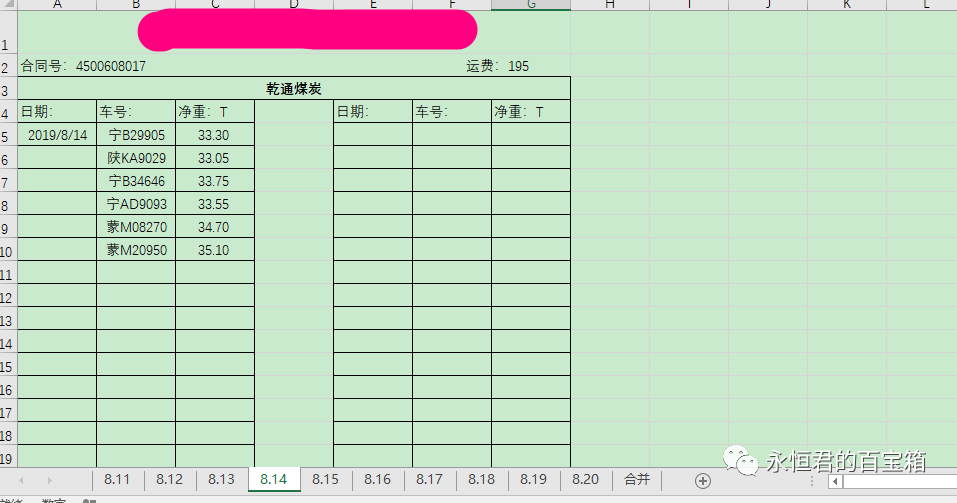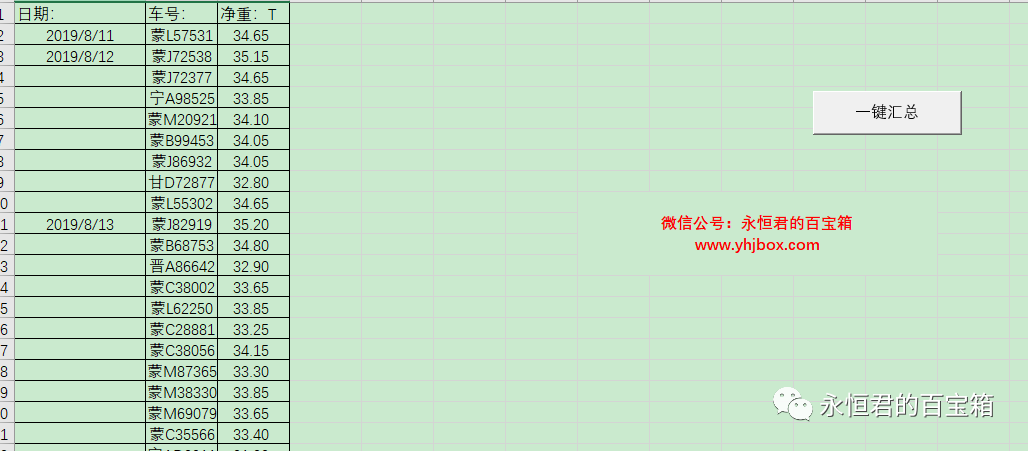1、每天的车辆数量不相同，有的日期多，有的日期少。
2、每天的sheet中，如果遇到车辆信息比较多的时候，信息会分成两列。

### 核心代码

``````  For Each sht In Worksheets #遍历每天的sheet
If sht.Name <> ActiveSheet.Name Then
For i = 5 To 100 #从第五行车辆信息开始，遍历sheet的每一行
If Len(sht.Range("B" & i)) > 0 Then
sht.Range("A" & i, "C" & i).Copy Cells(copy_hang, 1)
copy_hang = copy_hang + 1
End If
Next

For i = 5 To 100 #遍历sheet的另外一列车辆信息
If Len(sht.Range("F" & i)) > 0 Then
sht.Range("E" & i, "G" & i).Copy Cells(copy_hang, 1)
copy_hang = copy_hang + 1
End If
Next
End If
Next
``````

### 效果图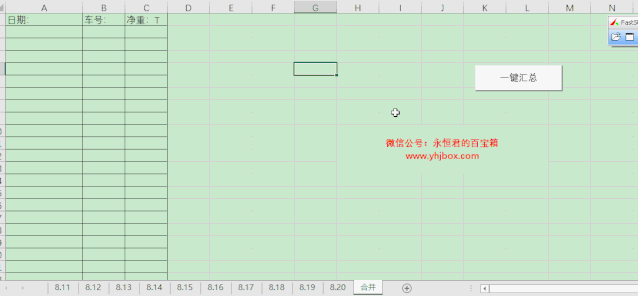### 拓展一下

``````For i = 5 To 100 #从第五行车辆信息开始，遍历sheet的每一行
``````

`````` start_hang = 5
Do While True
If Len(sht.Range("B" & start_hang)) > 0 Then
sht.Range("A" & start_hang, "C" & start_hang).Copy Cells(copy_hang, 1)
start_hang = start_hang + 1
copy_hang = copy_hang + 1
End If
If Len(sht.Range("B" & start_hang)) = 0 Then#如果车辆信息为空，则退出遍历循环
Exit Do
End If
Loop
``````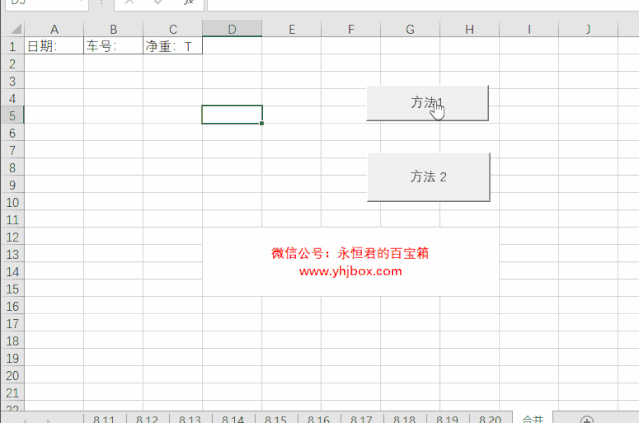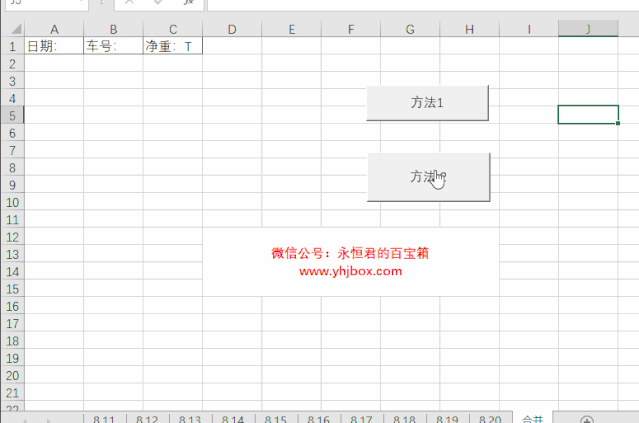Excel vba 实例(18) – 一键将单列长数据平均拆成多列收藏 | 17个Excel vba实例汇总

Excel vba 实例(17) – 遍历多个工作簿并提取内容到总表

Excel vba 实例(16) – 按指定字段分类批量提取内容

Excel vba 实例(15) – 按指定字段一键筛选并取最低价记录anyShare分享到：